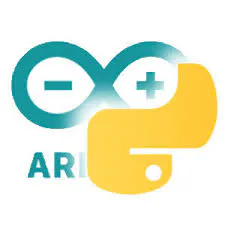# WW2 Tank Laser Tag Sherman & Panther

Create 2 tanks and battle them out, using laser light as the ammo!

## Things used in this project

### Hardware components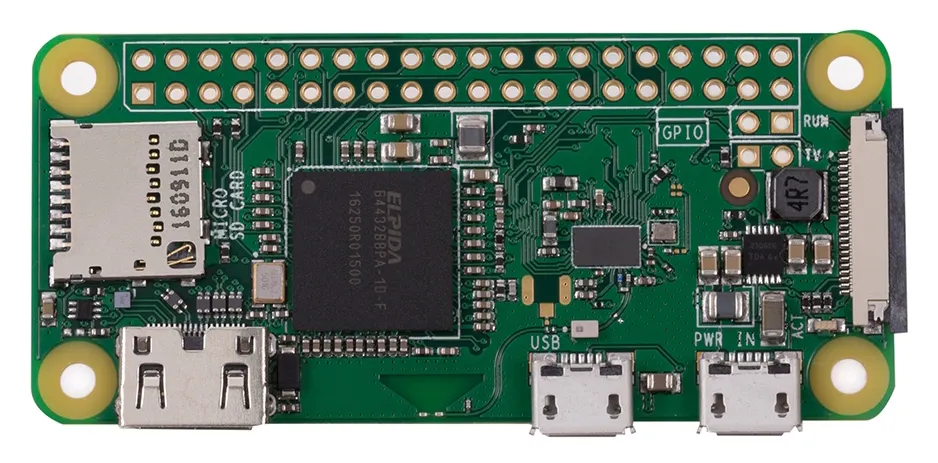Raspberry Pi Zero Wireless
×2
 DFRobot MiniQ Robot Chassis Kit
×2Texas Instruments Dual H-Bridge motor drivers L293D
×2
 Perf Board 6x4cm
×1
 5V Regulator
×4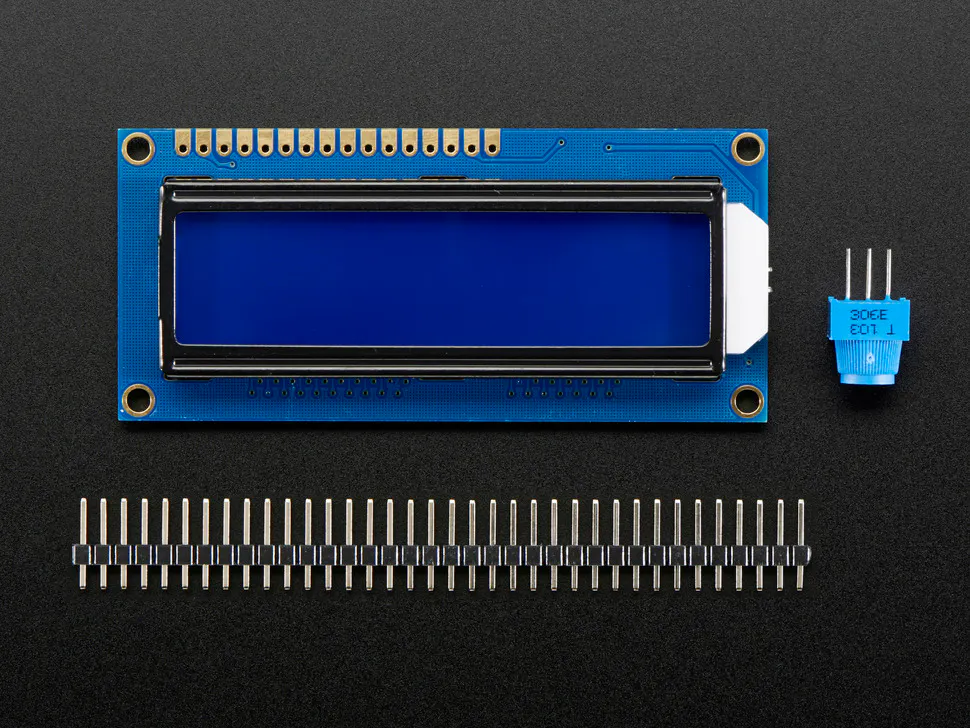Adafruit Standard LCD - 16x2 White on Blue
×2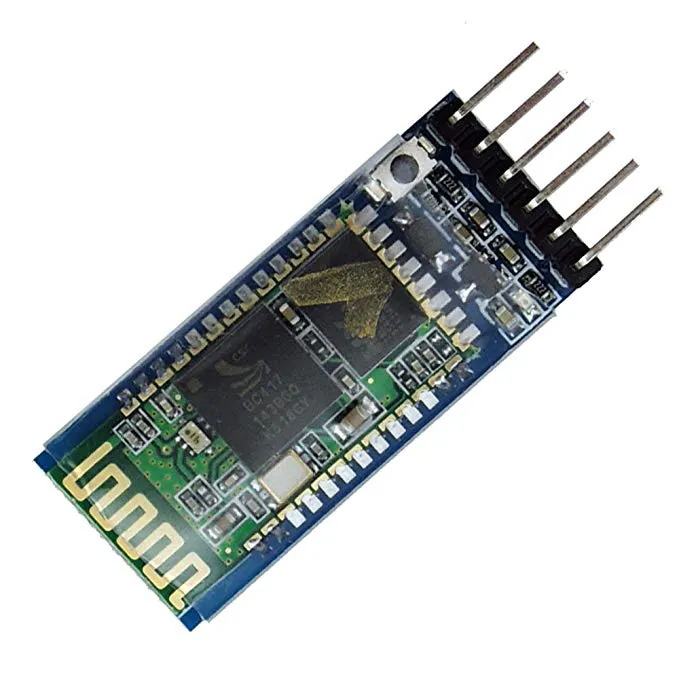HC-05 Bluetooth Module
×2
 Thumb Joystick
×4
×2LED (generic)
×10
 Laser Diode
×2
 Photoresistor
×8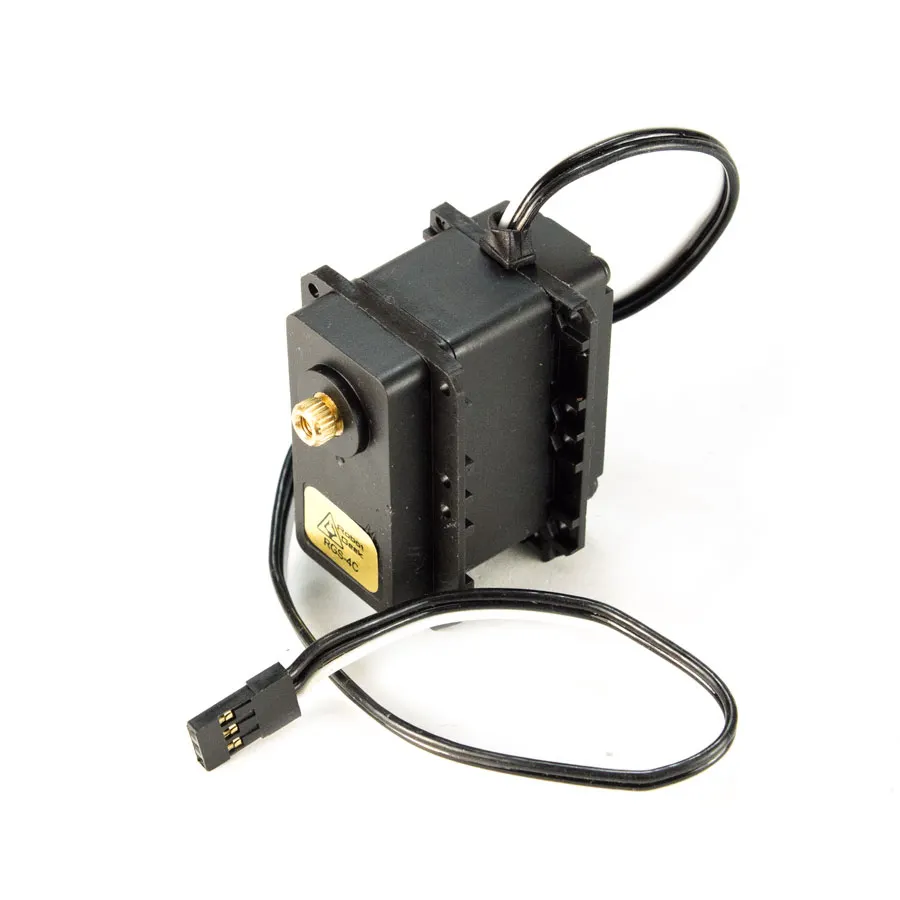RobotGeek Continuous Rotation Servo
×2
 Servo Micro 9g
×2
 1800 mAh LiPo Battery 11.1V 30C
×2
 1300 mAh LiPo Battery 7.4V 30C
×2SparkFun Pushbutton switch 12mm
×2
 Toggle Switch
×2
×2
 SPDT 2 Position 3 Terminal Switch
×1Arduino Nano R3
×2

### Software apps and online servicesArduino IDE

### Hand tools and fabrication machines3D Printer (generic)
 CNC RouterSoldering iron (generic)

## Code

### Robot Code Python Class

Python
```from __future__ import division
import RPi.GPIO as GPIO
from time import sleep
from serial import Serial
import json

class Car(object):

def __init__(self, BT_Port, laser_pin):
GPIO.setmode(GPIO.BCM)
self.ser = Serial(BT_Port, baudrate=9600,timeout=0)
self.laser_pin = laser_pin
self.laser_state = False
GPIO.setup(self.laser_pin, GPIO.OUT)

def configMotors(self, mL1, mL2, mR1, mR2, invertLR=False):
self.motor_array = [mL1, mL2, mR1, mR2]
self.invert = invertLR
for pin in self.motor_array:
GPIO.setup(pin, GPIO.OUT)
#self.motor_pwm.append(GPIO.PWM(pin, 490))
self.motorL1_pwm = GPIO.PWM(mL1, 100)
self.motorL2_pwm = GPIO.PWM(mL2, 100)
self.motorR1_pwm = GPIO.PWM(mR1, 100)
self.motorR2_pwm = GPIO.PWM(mR2, 100)
self.motorL1_pwm.start(0)
self.motorL2_pwm.start(0)
self.motorR1_pwm.start(0)
self.motorR2_pwm.start(0)

def configServos(self):
self.servo_min = 150
self.servo_max = 600
self.pwm.set_pwm_freq(60)

def deg_to_pulse(self, degree):
pulse = (degree - 0) * (self.servo_max - self.servo_min) / (180 - 0) + 150
print('Pulse: '+str(pulse))
return int(pulse)

self.cmd = ""
if  self.ser.inWaiting():
if self.cmd != None:
try:
data = self.cmd.decode('utf-8')
print data
if data != "":
return data
else:
return ""
except ValueError:
pass
#except IOError:
#pass

def turn_wheel(self, motor1, motor2):
if self.invert:
temp = motor1
motor1 = motor2
motor2 = temp
motor1 = (motor1 / 2)
motor2 = (motor2 / 2)
if motor1<0:
self.motorL2_pwm.ChangeDutyCycle(motor1*-1)
self.motorL1_pwm.ChangeDutyCycle(0)#make positive if negative
else:
self.motorL1_pwm.ChangeDutyCycle(motor1)
self.motorL2_pwm.ChangeDutyCycle(0)

if motor2<0:
self.motorR2_pwm.ChangeDutyCycle(motor2*-1)
self.motorR1_pwm.ChangeDutyCycle(0)#make positive if negative
else:
self.motorR1_pwm.ChangeDutyCycle(motor2)
self.motorR2_pwm.ChangeDutyCycle(0)

def move_turret(self, turret_deg, barrel_deg):
self.pwm.set_pwm(0, 0, self.deg_to_pulse(turret_deg))
self.pwm.set_pwm(1, 0, self.deg_to_pulse(barrel_deg))

def fire_laser(self, state):
GPIO.output(self.laser_pin, state)

def update(self): #Run to make the robot work
print self.json_obj
try:
if "motor" in self.cmd:
if self.json_obj["motor"] != None:
val1 = self.json_obj["motor"]
# if val1 > 0:
#    val1 += 10
val2 = self.json_obj["motor"]
# if val2 <0:
#   val2 += 4
self.turn_wheel(val1, val2)
elif "shoot" in self.cmd:
self.laser_state = self.json_obj["shoot"]
self.fire_laser(self.json_obj["shoot"])
elif "turret" in self.cmd:
val1 = self.json_obj["turret"]
val2 = self.json_obj["turret"]
self.move_turret(val1, val2)

except TypeError:
pass
sleep(.01)
```

### Robot Code Python Main

Python
```import car

bt_port = "/dev/rfcomm3"
laser_pin = 20
rc_car = car.Car(bt_port, laser_pin)

rc_car.configMotors(6,5,19,13, invertLR=True)
rc_car.configServos() #Turret drive servo, barrel servo

while 1:
rc_car.update()

```

### Controller Code

C/C++
```#include "Timer.h"
#include <Wire.h>
#include <LiquidCrystal_I2C.h>

int JS_VALS = {};
int JS_VALS_R = {};

const int JS_PINS = {A0, A1, A2, A3}; //L_X, L_Y, R_X, R_Y
const int led_pins = {4,5,6,7};

const int button_pin = 3;
bool laser = false;

const int servo_limits = {0, 180, 50, 95, 75}; //Left, Right, Lower, Upper, Middle limits for the turret
int turret_positions = {90, servo_limits}; //Initial positions

unsigned long last_time = 0;
long motor_delay = 30; //Delay 30 ms between motor control polls
long turret_delay = 150; //Delay 150 ms between turret control polls

float driveSpeed = 1.0;
int crewValues = {3,3,2,1};
bool crewLeft = {true,true,true,true};
int crewLeftNum = 4;
bool can_move = true;
bool can_fire = true;

LiquidCrystal_I2C lcd(0x3F, 16, 2);

Timer t;

void setup() {
// put your setup code here, to run once:
Serial.begin(9600);
lcd.init();
lcd.backlight();
lcd.print("Begin fighting!");
delay(2000);
lcd.clear();
lcd.home();
pinMode(button_pin, INPUT_PULLUP);
Serial.println("Begin");

t.every(motor_delay, control_motors);
t.every(turret_delay, control_turret);
t.every(50, checkButton);
for(int i=0;i<4;i++){
pinMode(led_pins[i], OUTPUT);
}
updateCrew();

}

void loop() {
/*control_motors();
control_turret();
delay(150);*/
t.update();
if(Serial.available()>0){
if(data=="HG"){
crewLeft = false;
crewLeftNum -= 1;
can_fire = false;
crewReplaceTimer = t.after(6000, replaceCrew);
}
else if(data=="HD"){
crewLeft = false;
crewLeftNum -= 1;
driveSpeed -= .2;
can_move = false;
crewReplaceTimer = t.after(6000, replaceCrew);
}
else if(data=="HC"){
crewLeft = false;
crewLeftNum -= 1;
driveSpeed -= .1;
crewReplaceTimer = t.after(6000, replaceCrew);
}
else if(data=="HM"){
crewLeft = false;
crewLeftNum -= 1;
crewReplaceTimer = t.after(6000, replaceCrew);
}
updateCrew();
}
if(crewLeftNum<=1){
lcd_clear();
lcd.print("You lose");
while(1);
}
}

void checkButton(){
fire_laser();
}
}

void replaceCrew(){
if(crewLeft==false){
if(crewLeft==true){
crewLeft = false;
crewLeft = true;
can_fire = true;
}
else if(crewLeft==true){
crewLeft = false;
crewLeft = true;
can_fire = true;
}
}
else if(crewLeft==false){
if(crewLeft==true){
crewLeft = false;
crewLeft = true;
can_move = true;
}
else if(crewLeft==true){
crewLeft = false;
crewLeft = true;
can_move = true;
}
}
updateCrew();
t.stop(crewReplaceTimer);
}

void updateCrew(){
for(int i=0;i<4;i++){
digitalWrite(led_pins[i],crewLeft[i]);
}
}

void lcd_clear(){
lcd.clear();
lcd.home();
}

void fire_laser(){
if(can_fire){
lcd.clear();
lcd.print("Firing laser");
String json_string = "{\"motor\":[" + String(0)+","+String(0)+"]}"; //Stop tank before firing
Serial.println(json_string);
laser = true;
fireTimer = t.after(2000, stopLaser);

}
}
}

void stopLaser(){
t.stop(fireTimer);
laser = false;
lcd_clear();
}

lcd_clear();
}

void control_motors(){
int x = map(JS_VALS, 0, 1023, -50, 50);
x = constrain(x, -100, 100);
int y = map(JS_VALS, 0, 1023, -50, 50);
y = constrain(y, -100, 100);
int motor1 = x+y;
int motor2 = y-x;
motor1 = constrain(motor1, -100, 100);
motor2 = constrain(motor2, -100, 100);
motor1 = 0;
}
motor2 = 0;
}

motor1 = int(float(motor1)*driveSpeed);
motor2 = int(float(motor2)*driveSpeed);

if(can_move==false){
motor1 = 0;
motor2 = 0;
}

String json_string = "{\"motor\":[" + String(motor1)+","+String(motor2)+"]}";
Serial.println(json_string);
String json_string2;
if(laser){
json_string2 = "{\"shoot\":1}";
Serial.println(json_string2);
}
else if(!laser){
json_string2 = "{\"shoot\":0}";
Serial.println(json_string2);
}
}

void control_turret(){
JS_VALS_R = analogRead(JS_PINS); //Get X values
JS_VALS_R = analogRead(JS_PINS); //Get Y values
int x = map(JS_VALS_R+10, 0, 1023, 5, -5);
x = constrain(x, -5, 5); //Catch outliers
int y = map(JS_VALS_R, 0, 1023, 2, -2);
y = constrain(y, -2, 2);

turret_positions += x;
turret_positions += y;

if(turret_positions <= servo_limits){
turret_positions = servo_limits;
}
else if(turret_positions >= servo_limits){
turret_positions = servo_limits;
}
if(turret_positions <= servo_limits){
turret_positions = servo_limits;
}
else if(turret_positions >= servo_limits){
turret_positions = servo_limits;
}

String json_string = "{\"turret\":[" + String(turret_positions)+","+String(turret_positions)+"]}";
Serial.println(json_string);

}
```

## Credits

### Arduino “having11” Guy

32 projects • 879 followers
20 year-old IoT and embedded systems enthusiast. Also produce content for Hackster.io and love working on projects and sharing knowledge.
Thanks to AceofAces16.